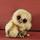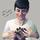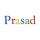## General Question# How do I find fixed cost and total variable cost?

Asked by chelle21689 (7706) August 1st, 2011

I’m so confused! Help…
Rooms Cleaned
0 3 7 12 16 17
Total Cost
100 200 300 400 500 600

Okay so I know the first total fixed cost for 0 is 100…but tten what??

Observing members: 0Composing members: 0## 3 AnswersI’m trying to answer as a person skilled in math, not economics, so if I use any false premises here due to ignorance of economics, someone please jump in and correct me!

Okay, so it seems to me that there is a particular amount charged before any work is done at all. I would assume that is what is meant by a fixed cost. It seems you’ve figured out the fixed cost already: it costs \$100 to clean 0 rooms, so \$100 must be the charge accumulated before any work is done, or the fixed cost. That’s a good start.

Look at the next set of numbers. \$200 for 3 rooms. You know that \$100 of that is the fixed cost, the amount charged before any work is completed at all. So the other \$100 is the price of the work of three rooms, or the variable cost. Can you use this information to determine what the total variable cost is?

Again someone please correct me if my ignorance of economics is causing me to go about this incorrectly. Really sorry if I’m leading you astray; just answering using my mathematical intuition.

Mariah (25876)“Great Answer” (0) Flag as…See this graph
If you plot such a graph with rooms on x axis & total costs on y axis, you will get a similar graph. Now,
Total cost = Fixed Cost + Variable Cost
That means, fixed cost is same for all rooms. Draw a horizontal line parallel to x axis, from the first point, which in this case is minimum. This is fixed cost. For the concerned example, it comes out to be 100.
You can get variable cost for each room by subtracting fixed cost from total cost for each room.

Total fixed cost is addition of fixed costs for all rooms; which comes as 600 (since 6 rooms & each room costs 100).
Total variable cost can be calculated by :
1) taking addition of variable costs of all rooms, or
2) subtracting total fixed cost from total of total costs (2100 – 600 = 1500), which is easier in this case.

prasad (3859)“Great Answer” (0) Flag as…Oh, sorry. I took it as room numbers, and not number of rooms.

prasad (3859)“Great Answer” (0) Flag as…## Answer this questionor

#### Join

to answer.

This question is in the General Section. Responses must be helpful and on-topic.

Your answer will be saved while you login or join.
Have a question? Ask Fluther!
What do you know more about?
or
Knowledge Networking @ Fluther